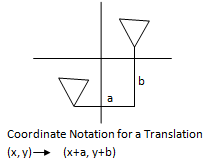Email us to get an instant 20% discount on highly effective K-12 Math & English kwizNET Programs!

#### Online Quiz (WorksheetABCD)

Questions Per Quiz = 2 4 6 8 10

### Geometry7.4 Translation

 Translation Mapping: A mapping of a plane in which each point of the plane moves in the same direction by the segments, all congruent to a given segment (equal distance) is called a translation. Translation moves every point of a figure the same distance in the same direction.Examples: Find the coordinates of the image of (2,7), under the translation defined by (x,y) ® (x+2, y+1). T(x,y) ® (x+2, y+1). T(2,7) ® (2+2, 7+1). The coordinates of the image is (4,8) A quadrilateral has vertices A(-4,3), B(-2,4), C(-1,1) and D(-3,1). Find the coordinates of the image after translation (x,y) ® (x+5,y-2). (x,y) ® (x+2, y+1). A(-4,3) ® (1, 1) B(-2,4) ® (3, 2) C(-1,1) ® (4, -1) D(-3,1) ® (2, -1) Directions: Choose the correct answer. Also write at least 5 examples of your own.
 Q 1: Find the coordinates of the image of (5, 6), under the translation defined by (x,y) ® (x+2, y-1).(7, 5)none(5, 7) Question 2: This question is available to subscribers only!

#### Subscription to kwizNET Learning System offers the following benefits:

• Unrestricted access to grade appropriate lessons, quizzes, & printable worksheets
• Instant scoring of online quizzes
• Progress tracking and award certificates to keep your student motivated
• Unlimited practice with auto-generated 'WIZ MATH' quizzes